# McGraw Hill Math Grade 6 Lesson 3.1 Answer Key Dividing Whole Numbers

Practice questions available in McGraw Hill Math Grade 6 Answer Key PDF Lesson 3.1 Dividing Whole Numbers will engage students and is a great way of informal assessment.

## McGraw-Hill Math Grade 6 Answer Key Lesson 3.1 Dividing Whole Numbers

Exercises
Divide

Question 1.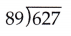Dividing 627 by 89, we get the quotient 7.04.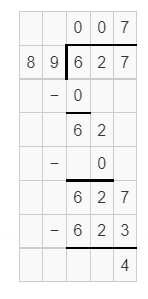Explanation:
627 ÷ 89 = 7.04.

Question 2.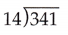Dividing 341 by 14, we get the quotient 24.35.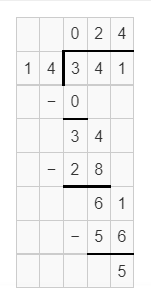Explanation:
Division of 341 ÷ 14 = 24.35.

Question 3.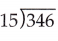Dividing 346 by 15,we get the quotient 23.06.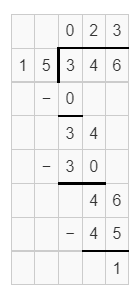Explanation:
Division of 346 ÷ 15 = 23.06.

Question 4.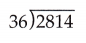Dividing 2814 by 36, we get the quotient 78.16.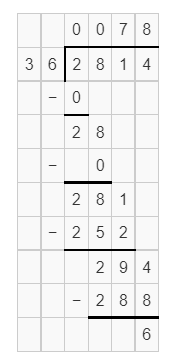Explanation:
Division of 2814 ÷ 36 = 78.16.

Question 5.Dividing 182 by 12, we get  the quotient 15.16.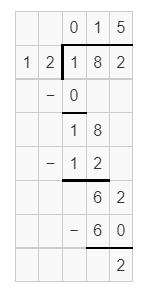Explanation:
Division of 182 ÷ 12 = 15.16.

Question 6.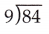Dividing 84 by 9, we get the quotient 9.33.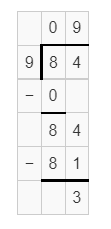Explanation:
Division of 84 ÷ 9 = 9.33.

Question 7.Dividing 7499 by 96, we get the quotient 78.11.Explanation:
Division of 7499 ÷ 96 = 78.11.

Question 8.Dividing 22220 by 18, we get the quotient 1234.44.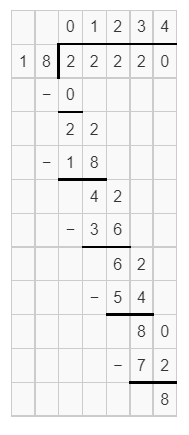Explanation:
Division of 22220 ÷ 18 = 1234.44.

Question 9.Dividing 17330 by 38, we get the quotient 456.05.Explanation:
Division of 17330 ÷ 38 = 456.05.

Question 10.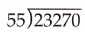Dividing 23270 by 55, we get the quotient 423.09.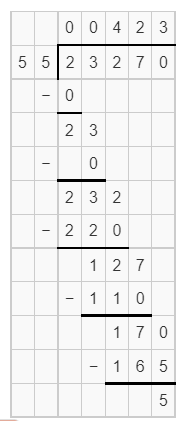Explanation:
Division of 23270 ÷ 55 = 423.09.

Question 11.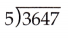Dividing 3647 by 5, we get the quotient 729.4.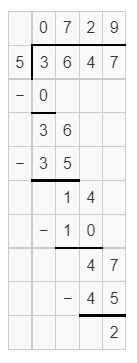Explanation:
Division of 3647 ÷ 5 = 729.4.

Question 12.Dividing 1399 by 82, we get the quotient 17.06.Explanation:
Division of 1399 ÷ 82 = 17.06.

Question 13.Dividing 6621 by 45, we get the quotient 147.13.Explanation:
Division of 6621 ÷ 45 = 147.13.

Question 14.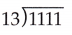Dividing 1111 by 13, we get the quotient 85.46.Explanation:
Division of 1111 ÷ 13 = 85.46.

Question 15.Dividing 257 by 14, we get the quotient 18.35.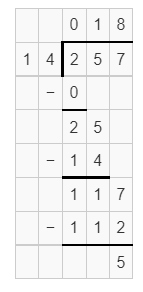Explanation:
Division of 257 ÷ 14 = 18.35.

Question 16.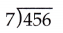Dividing 456 by 7, we get  the quotient 65.14.Explanation:
Division of 456 ÷ 7 = 65.14.

Question 17.Dividing 803 by 9, we get the quotient 89.22.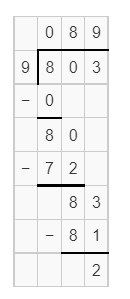Explanation:
Division of 803 ÷ 9 = 89.22.

Question 18.Dividing 499 by 11, we get the quotient 45.36.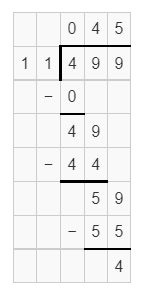Explanation:
Division of 499 ÷ 11 = 45.36.

Question 19.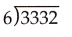Dividing 3332 by 6, we get the quotient 555.33.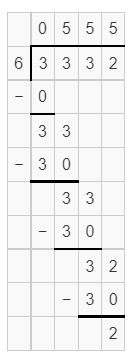Explanation:
Division of 3332 ÷ 6 = 555.33.

Question 20.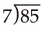Dividing 85 by 7, we get the quotient 12.14.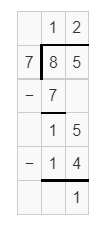Explanation:
Division of 85 ÷ 7 = 12.14.

Question 21.Dividing 157 by 12, we get the quotient 13.08.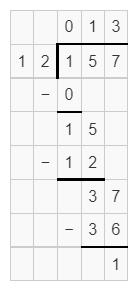Explanation:
Division of 157 ÷ 12 = 13.08.

Question 22.Dividing 3944 by 7, we get the quotient 563.42.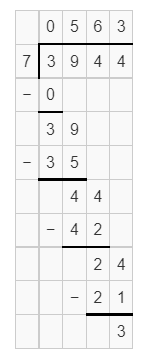Explanation:
Division of 3944 ÷ 7 = 563.42.

Question 23.Dividing 1115 by 4, we get the quotient 278.75.Explanation:
Division of 1,115 ÷ 4 = 278.75.

Question 24.Dividing 1517 by 17, we get the quotient 89.23.Explanation:
Division of 1,517 ÷ 7 = 89.23.

Question 25.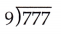Dividing 777 by 9, we get the quotient 86.33.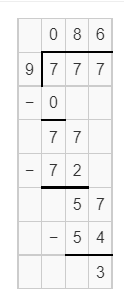Explanation:
Division of 777 ÷ 9 = 86.33.

Question 26.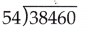Dividing 38460 by 54, we get  the quotient 712.22.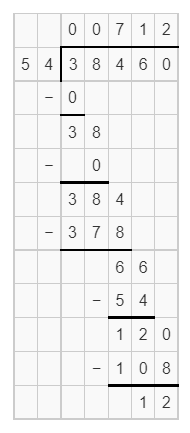Explanation:
Division of 38460 ÷ 54 = 712.22.

Question 27.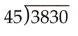Dividing 3830 by 45, we get the quotient 85.11.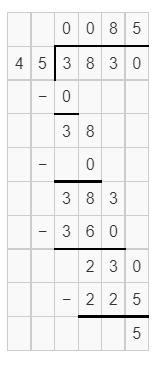Explanation:
Division of 3,830 ÷ 45 = 85.11.

Question 28.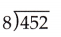Dividing 452 by 8, we get the quotient 56.5.Explanation:
Division of 452 ÷ 8 = 56.5.

Question 29.
Post office workers are placing envelopes in boxes. Each worker can place 625 envelopes per day. How many days will it take one worker to place 2,500 envelopes?
Number of days it takes one worker to place all envelopes = 4.

Explanation:
Number of envelopes each worker can place per day = 625.
Number of envelopes = 2,500.
Number of days it takes one worker to place all envelopes = Number of envelopes ÷ Number of envelopes each worker can place per day
= 2,500 ÷ 625
= 4.

Question 30.
Mr. Romanski is making information packets for all his new students. Each packet is 19 pages long. If he uses 342 sheets of paper, how many new students are in his class?